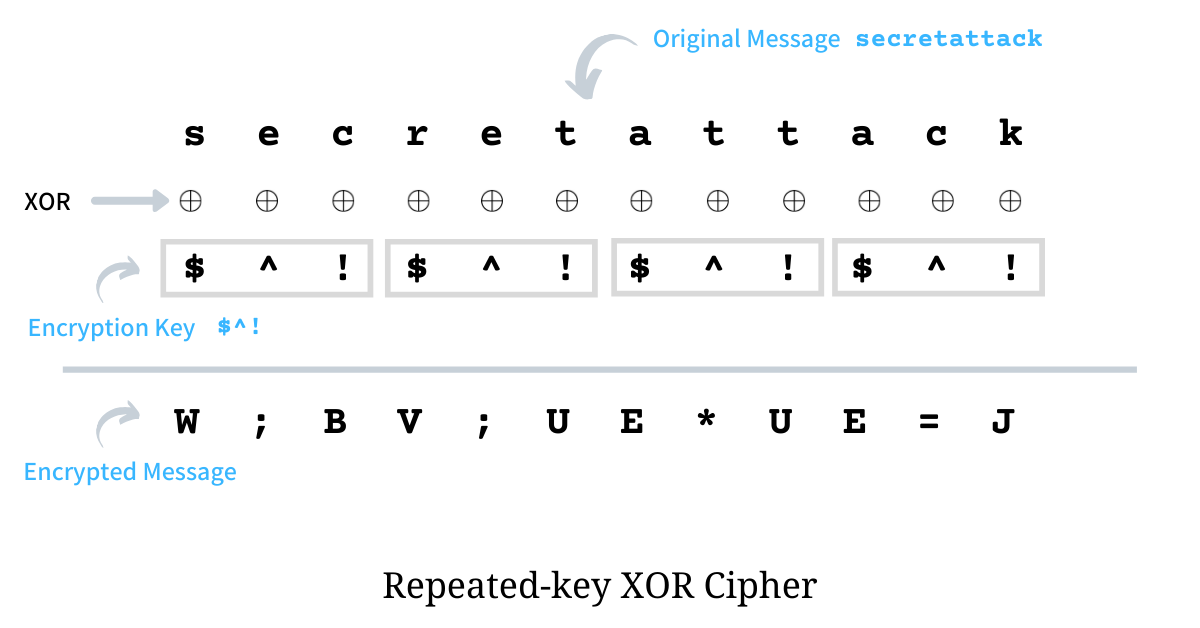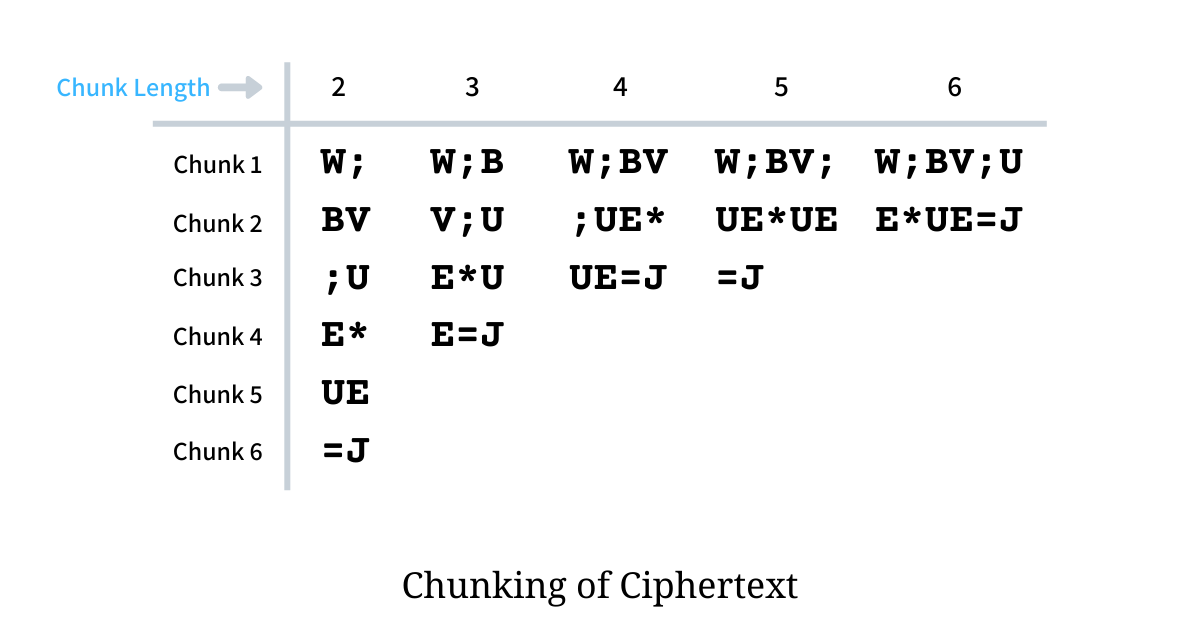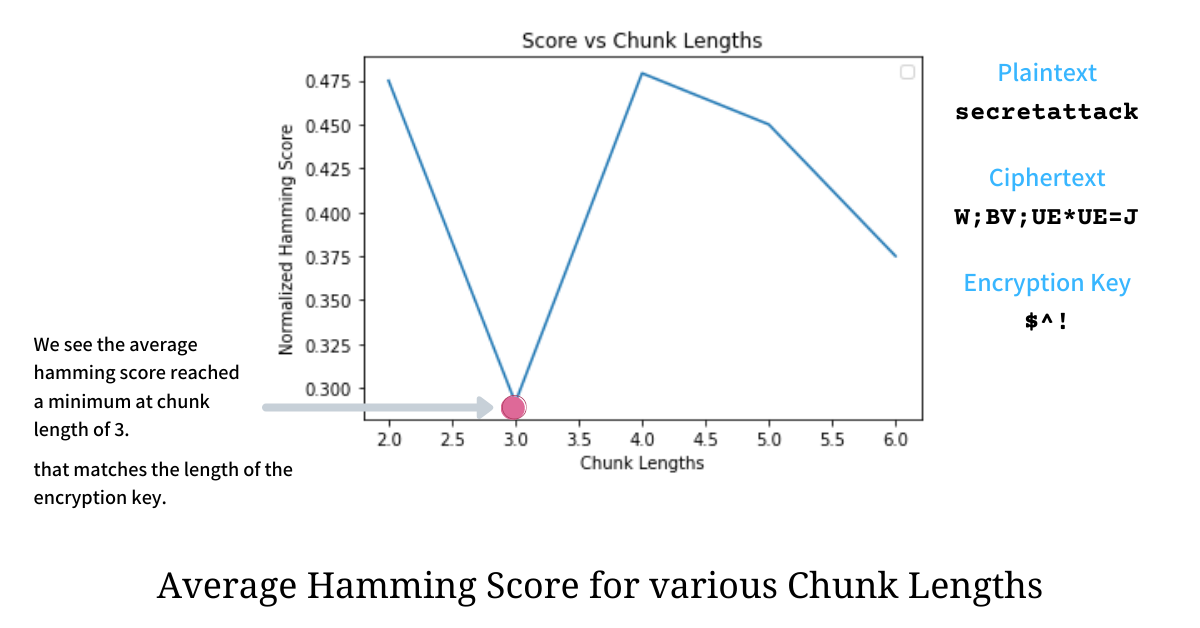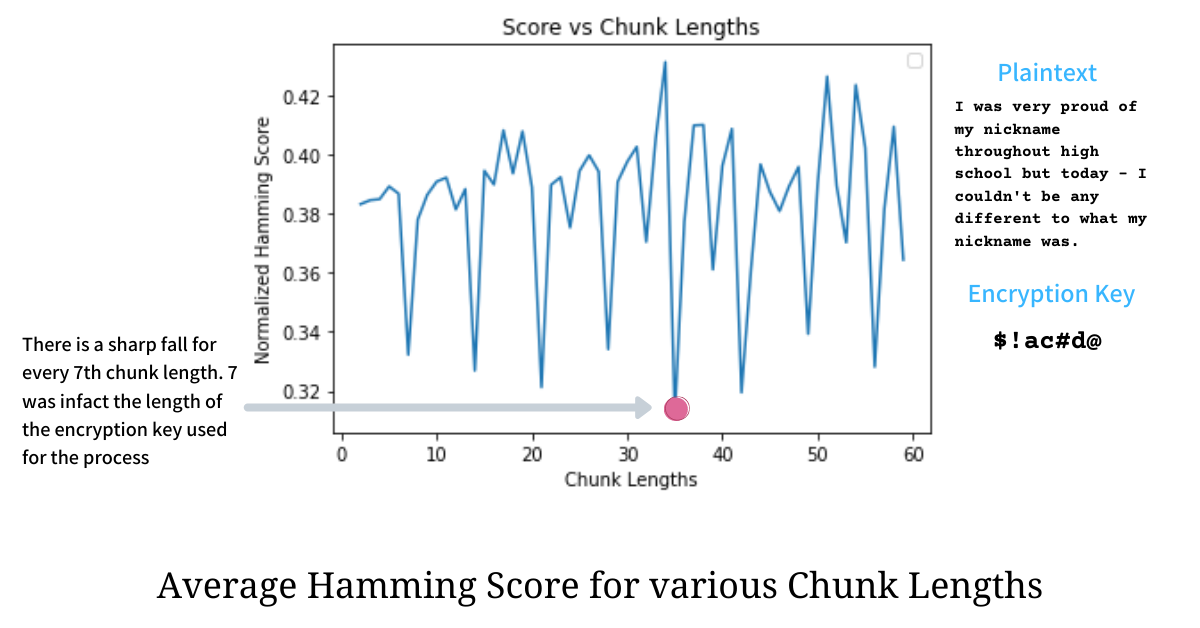# Deciphering Repeated-key XOR Ciphertext

Encryption is a process of encoding messages such that it can only be read and understood by the intended parties. The process of extracting the original message from an encrypted one is called Decryption. Encryption usually scrambles the original message using a key, called the encryption key, that the involved parties agree on.

In the previous essay, we went through the Single-byte XOR cipher and found a way to decipher it without having any knowledge of the encryption key. In this essay, we find how to break a Repeating-key XOR cipher with variable key length. The problem statement, defined above, is based on Cryptopals Set 1 Challenge 6.

# Repeating-key XOR Cipher

The Repeating-key XOR cipher algorithm works with an encryption key with no constraint on its length, which makes it much stronger than a Single-byte XOR Cipher, where the encryption key length was restricted to a single byte.

## Encryption

A plain text is encrypted using an encryption key by performing a bitwise XOR operation on every character. The encryption key is repeated until it XORs every single character of the plain text and the resultant stream of bytes is again translated back as characters and sent to the other party. These encrypted bytes need not be among the usual printable characters and should ideally be interpreted as a stream of bytes. Following is the python-based implementation of this encryption process.

``````def repeating_key_xor(text: bytes, key: bytes) -> bytes:
"""Given a plain text `text` as bytes and an encryption key `key`
as bytes, the function encrypts the text by performing
XOR of all the bytes and the `key` (in repeated manner) and returns
the resultant XORed byte stream.
"""

# we update the encryption key by repeating it such that it
# matches the length of the text to be processed.
repetitions = 1 + (len(text) // len(key))
key = key * repetitions
key = key[:len(text)]

# XOR text and key generated above and return the raw bytes
return bytes([b ^ k for b, k in zip(text, key)])``````

As an example, we encrypt the plain text - `secretattack` - with encryption key `\$^!` and as per the algorithm, we first repeat the encryption key until it matches the length of the plain text and then XOR it against the plain text. The illustration below shows the entire encryption process.For the first character in plain text - `s` - the byte i.e. ASCII value is `115` which when XORed with `\$` results in `87` whose character equivalent is `W`, similarly for the second character `e` the encrypted byte is `;`, for `c` it is `B`, for the fourth character `r`, since the key repeats, the XOR is taken with `\$` to get `V` and the process continues. The resultant encrypted text using repeated-key XOR on the plain text `secretattack` with key `\$^!` is `W;BV;UE*UE=J`.

## Decryption

Decryption is a process of extracting the original message from the encrypted ciphertext given the encryption key. XOR has a property - if `a = b ^ c` then `b = a ^ c`, hence the decryption process is exactly the same as the encryption i.e. we first repeat the encryption key till it matches the length and then perform bitwise XOR with the ciphertext - the resultant bytes stream will be the original message.

Since encryption and decryption both have an exact same implementation - we pass the ciphertext to the function `repeating_key_xor`, defined above, to get the original message back.

``````>>> repeating_key_xor(b'W;BV;UE*UE=J', b'\$^!')
b'secretattack'``````

# Deciphering without the encryption key

Things become really interesting when, given the encryption algorithm, we have to recover the original message from the ciphertext with no knowledge of the encryption key. Just like solving any other problem, the crux of deciphering the message encrypted using repeated-key XOR cipher is to break it down into manageable sub-problems and tackle them independently. We break this deciphering problem into the following two sub-problems:

• Finding the length of the Encryption Key
• Bruteforce with all possible keys and finding the “most English” plain text

## Finding the length of the Encryption Key

In order to recover the original text from the cipher, we first find the length of the encryption key used and then apply brute force with all possible keys of the estimated length and deduce the plain text. Finding the length of the Encryption key makes the deciphering process quicker as it eliminates a lot of false keys and thus reducing the overall effort required during the brute force. In order to find the length of the Encryption Key, we need to have a better understanding of a seemingly unrelated topic - Hamming Distance.

### Hamming Distance

Hamming distance between two bytes is the number of positions at which the corresponding bits differ. For a stream of bytes, of equal lengths, it is the sum of Hamming Distances between the corresponding bytes. Finding differences between bits can be efficiently done using bitwise XOR operation as the operation yields `0` when both the bits are the same and `1` when they differ. So for computing Hamming Distance between two bytes we XOR the bytes and count the number of `1` in its binary representation.

``````def hamming_distance_bytes(text1: bytes, text2: bytes) -> int:
"""Given two stream of bytes, the function returns the Hamming Distance
between the two.
Note: If the two texts have unequal lengths, the hamming distance is
computed only till one of the text exhausts, other bytes are not iterated.
"""
dist = 0
for byte1, byte2 in zip(text1, text2):
dist += bin(byte1 ^ byte2).count('1')
return dist

>>> hamming_distance_bytes(b'ab', b'zb')
4``````

In the example above, we find that the hamming distance between two bytestreams `ab` and `zb` is `4`, which implies that the byte streams `ab` and `zb` differ at `4` different bits in their binary representations.

### Hamming Score

Hamming distance is an absolute measure, hence in order to compare hamming distance across byte streams of varying lengths, it has to be normalized with the number of pairs of bits compared. We name this measure - Hamming Score - which thus is defined as the Hamming Distance per unit bit-pair. In python, Hamming Score is implemented as:

``````def hamming_score_bytes(text1: bytes, text2: bytes) -> float:
"""Given two streams of bytes, the function computes a normalized Hamming
Score based on the Hamming distance.
Normalization is done by dividing the Hamming Distance by the number of bits
present in the shorter text.
"""
return hamming_distance_bytes(text1, text2) / (8 * min(len(text1), len(text2)))

>>> hamming_score_bytes(b'ab', b'zb')
0.25``````

### What can we infer through Hamming Distance?

Hamming Distance is an interesting measure; it effectively tells us the minimum number of bit flips required to convert one bytestream into another. It also implies that (on average) if the numerical values of two bytestreams are closer then their Hamming Distance and Hamming Score will be lower i.e it would take fewer bit flips to convert one into another.

This is evident from the fact that the average Hamming distance between any two bytes `[0-256)` picked at random is `3.9999` while that of any two lowercased English characters `[97, 122]` is just `2.45`. Similar ratios are observed for Hamming Score where `0.4999` is of the former while `0.3072` is of the later.

This inference comes in handy when we want to find out the length of Encryption Key in Repeating-key XOR Cipher as illustrated in the section below.

### Formal definition of encryption and decryption processes

Say if `p` denotes the plaintext, `k` denotes the encryption key which is repeated to match the length of the plain text, and `c` denotes the ciphertext, we could define encryption and decryption processes as

``````encryption: c[i] = p[i] XOR k[i]   for i in [0, len(c))
decryption: p[i] = c[i] XOR k[i]   for i in [0, len(p))``````

The above definitions, along with the rules of XOR operations, implying that if we XOR two bytes of the ciphertext, encrypted (XORed) using the same byte of the encryption key, we are effectively XORing the corresponding bytes of the plain text. If `k'` is the byte of the encryption key `k` which was used to encrypt (XOR) the bytes `p[i]` and `p[j]` of the plain text to generate `c[i]` and `c[j]` of the ciphertext, we could derive the following relation

``````# k' is the common byte of the key i.e. k' = k[i] = k[j]

c[i] XOR c[j] = (p[i] XOR k') XOR (p[j] XOR k')
= p[i] XOR k' XOR k' XOR p[j]
= p[i] XOR 0 XOR p[j]
= p[i] XOR p[j]``````

The above relation, `c[i] XOR c[j]` equal to `p[i] XOR p[j]`, holds true only because both the bytes were XORed with the same byte `k'` of the encryption key; which in fact helped reduce the expression. If the byte from the encryption key which was used to XOR the pain texts were different then the relation was irreducible and we could not have possibly setup this relation.

### Chunking of ciphertext

Chunking is the process where the ciphertext is split into smaller chunks (segments) of almost equal lengths. For example, chunking the ciphertext `W;BV;UE*UE=J` for chunk length `4` would create `3` chunks `W;BV`, `;UE*` and `UE=J`. The illustration below shows the chunks that would be formed for `W;BV;UE*UE=J` with chunks lengths varying from 2 to 6.### XOR of the chunks

Something very interesting happens when we compute the Average Hamming Score for all possible chunk lengths. If we consider the ciphertext `b'W;BV;UE*UE=J` and we chunk it with lengths varying from 2 to 6, we get the following distribution for the Average Hamming Score for each of the chunk length.From the distribution above it is evident that the score was minimum at chunk length equalling `3`, which actually was the length of the Encryption Key used on the plain text. Is this mere coincidence or are we onto something?

When chunk length is equal to the length of the encryption key, the XOR operation on any two chunks will reduce the expression to XOR of the corresponding plain texts (as seen above), because there will be a perfect alignment of bytes from ciphertext and bytes from the keys i.e every `i`th byte from both the chunks would have been XORed with `i`th byte from the encryption key.

We have established that for chunk length equal to the length of the encryption key `c[i] XOR c[j]` is effectively `p[i] XOR p[j]`. Since we have assumed that the plain text is a lowercased English sentence the XOR is happening between bytes residing numerically closer to each other and hence has a lower Average Hamming Score between them; because of which we see a minimum at this particular chunk length. The Hamming Score will be much higher for lengths other than the length of Encryption Key because during XOR operation the expression stays irreducible and hence hamming distance is computed panning the entire range of bytes `[0, 256)`.

### Something far more interesting

This minimum does not only hold true for chunk length equal to the length of the encryption key, but it also holds true when the length of the chunk is a multiple of the length of the encryption key. This happens because for repeated keys when the chunk length is a multiple of Encryption Key there will be a perfect alignment of bytes such that every `i`th byte of chunks is XORed with `i`th byte of the encryption key; which sets up the relation `c[i] XOR c[j]` equalling `p[i] XOR p[j]`.The above distribution shows a lot of sharp drops of Average Hamming Score for chunk lengths that are multiples of `7` - the length of the encryption key used.

## Computing Encryption Key Length

Now that we understand the theory and concept behind the process of finding the length of the Encryption Key, we can compile the logic into a function that accepts `text` and bytes and returns the length of the Encryption Key as illustrated below

``````def compute_key_length(text: bytes) -> int:
"""The function returns the length of the encryption key
by chunking and minimizing the Average Hamming Score
"""
min_score, key_len = None, None

# We check for chunk lengths from 2 till the half the length of the
# plain text. Here we assume that the Encryption Key had to be
# repeated at least twice to match the length of the plaintext
for klen in range(2, math.ceil(len(text)/2)):

# We create chunks such that length of each chunk if `klen`
chunks = [
text[i: i+klen]
for i in range(0, len(text), klen)
]

# To gain better accuracy we get rid of the last chunk that had
# length smaller than klen/2
if len(chunks) >= 2 and len(chunks[-1]) <= len(chunks[-2])/2:
chunks.pop()

# For each chunk length, for every pair of chunks we compute the
# Hamming Score and keep piling it in a list.
_scores = []
for i in range(0, len(chunks) - 1, 1):
for j in range(i+1, len(chunks), 1):
score = hamming_score_bytes(chunks[i], chunks[j])
_scores.append(score)

# The Hamming Score for a chunk length is the average
# hamming score computed over all possible pairs of chunks
score = sum(_scores) / len(_scores)

# Keep track of the minimum score we have seen and the key length
# corresponding to it.
if min_score is None or score < min_score:
min_score, key_len = score, klen

# return the key length corresponding to the minimum score
return key_len``````

## Bruteforce to recover the original text

The function `compute_key_length` returns the length of the Encryption Key used to encrypt the plain text. Once we know the length, we can apply Bruteforce with all possible keys of that length and try to decipher the ciphertext. The approach of deciphering will be very similar to how it was done to Decipher single-byte XOR Ciphertext i.e. by using Letter Frequency Distribution and Fitting Quotient to find which key leads to the plain text that is closest to a genuine English sentence.

A test was run on 100 random English sentences with random Encryption keys of varying lengths and it was found that this deciphering technique worked with an accuracy of 99%. Even though the approach is not fool-proof, it does pretty well in eliminating keys that would definitely not result in a correct plain text.

# Conclusion

Deciphering a repeated-key XOR Cipher could also be done using Kasiski examination; the method we saw in this essay was Friedman Test using Hamming Distance and Frequency Analysis. The main purpose of this essay was to showcase how seemingly unrelated concepts work together to solve an interesting problem efficiently.

### Courses

Super practical courses, with a no-nonsense approach, are designed to spark engineering curiosity and help you ace your career.

## System Design for Beginners

An in-depth, self-paced, and on-demand course that for early engineers to become great at designing scalable, available, and extensible systems at scale.

## System Design Masterclass

A masterclass that helps experienced engineers become great at designing scalable, fault-tolerant, and highly available systems.

## Redis Internals

A course that helps covers Redis internals by reimplementing its core features like - event loop, serialization protocol, pipelining, eviction, and transactions.## CS newsletter for the curious engineers

If you like what you read subscribe you can always subscribe to my newsletter and get the post delivered straight to your inbox. I write essays on various engineering topics and share it through my weekly newsletter.

Writings and Videos
Legal and Contact
Everything Else